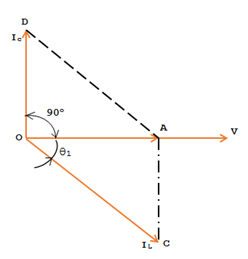How Synchronous Motor Used for Power Factor Correction

The power factor of a synchronous motor is changed with a change in the excitation. When the excitation of the motor is increased, the power factor changes from lagging to unity and then to a leading power factor. This property of the motor is utilized to improve the power factor of the leads, having a low lagging power factor. Normally, when the motor is utilized in this way to improve the factor, the synchronous motor is run without any mechanical load. The excitation is adjusted in such a manner that it works at a leading power factor. The synchronous motor is then referred to a synchronous condenser.

In factories the majority of the motors used are induction motors, the full load power factor of these motor may be around 0.8 lagging. The power factor of these motors may operate at full load and many of them operate at light loads. This results in the power factor of the installation reading as low as 0.6 lagging. A synchronous condenser may be connected in parallel with the induction motors. This will improve the power factor of the installation.

Synchronous Phase Modifier:

For controlling the voltage of a transmission line, at the receiving end, a synchronous motor is used. In this application, the synchronous motor is made to run on no load and draw a leading current. The synchronous motor when used for the above purpose is called synchronous phase modifier. As the power factor of the system is improved by the action of synchronous condenser, the drop of volts from the sending to receiving end on the line is decreased and the regulation of the line is improved.

% regulation = {(E-V)/V} x 100

Power Factor Correction with Synchronous Condenser:

The power factor of a load can be improved to unity or desired value from cosθ1 to cosθ2 by installing and operating a synchronous condenser in the system. The losses in the motor can be neglected and the motor will reactive current leading by 90o. The figure shows the phasor diagram for the operation of a synchronous motor to improve the power factor.

Synchronous Motor Used for Power factor Correction :In the above figure, V is the reference phasor IC is the load current of power factor cosθ1, lagging. OA is the active component of load current and AC is the reactive component. If the synchronous motor is run as synchronous condenser and losses are neglected OD represents the current taken, it leading by 90o. If this is made equal to the reactive load current component AC, the resultant of the currents drawn by load and the synchronous motor will be OA only, giving the same power output in KW to load but improving the power factor of load to unity as OA is in phase with V.

Thus Ic = ILsinθ1 is the condition to improve the power factor of the load to unity under given operating condition.

The KVA rating of the synchronous condenser required for the purpose is Ic x V volt amps per phase or  √3VIc/1000 KVA would be the three phase rating.

The rating of the synchronous condenser is √3VIc/1000 KVA. If it is desired to improve the power factor less than unity the capacity of synchronous condenser required will be less.

In the fig cosθ is the initial power factor of the load:
OA – ILcosθ1 is the active component of the current
AC – ILsinθ1 is the reactive component of load current (Lagging)
OD – reactive (leading) component of the synchronous condenser

If it is made equal to BC, then the resultant load current phasor is OB and the new power factor of the load is cosθ2. Then
Ic = ILsinθ1 – ILsinθ2

The active component of load gets reduced from AC to AB. The synchronous condenser rating required for the purpose is
= √3VIc/1000 KVA

The power component of load OA remaining the same and the same load is supplied but now at improved power factor2 CommentsComments

1.Are synchronous motors or capacitors more effective for power factor correction in factories mainly using induction motors and can a generator driven by a synchronous alternator suitable

2.Are synchronous motors or capacitors more effective for power factor correction in factories mainly using induction motors and can a generator driven by a synchronous alternator suitable